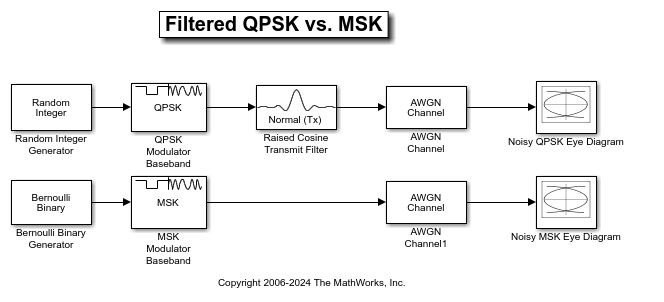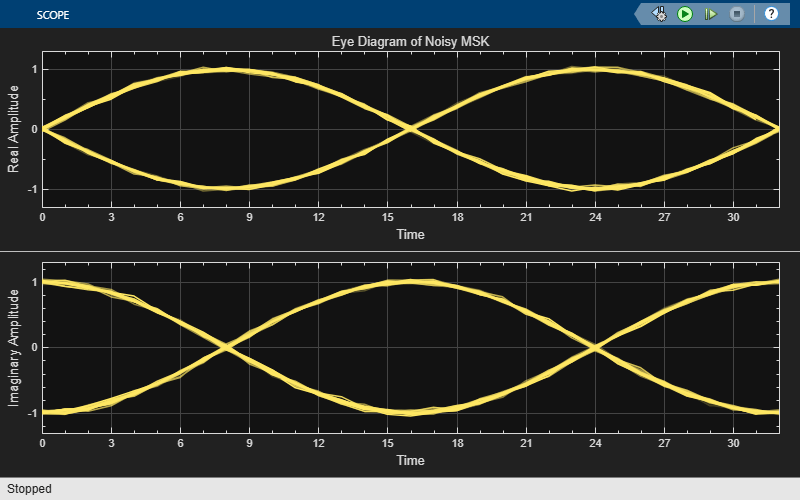# Compare Filtered QPSK and MSK Signals in Simulink

This model compares filtered quadrature phase shift keying (QPSK) and minimum shift keying (MSK) modulation schemes.The model generates the filtered QPSK signal using random integer data from the Random Integer Generator block, which gets modulated by the QPSK Modulator Baseband block, and then filtered by the Raised Cosine Transmit Filter block. The model generates the MSK signal using random binary data from the Bernoulli Binary Generator block, which gets modulated by the MSK Modulator Baseband block. Noise is added to both the filtered QPSK and MSK signals by using AWGN Channel blocks. The Eye Diagram Scope blocks are used to visualize eye diagrams of both signals.

For filtered QPSK modulation, the values of both the in-phase and quadrature components of the signal are permitted to change at any symbol interval. For MSK modulation, the symbol interval is half that for QPSK, but the in-phase and quadrature components change values in alternate symbol epochs.

Compare eye diagram plots of a QPSK modulated signal and an MSK modulated signal. For QPSK the ideal sampling period is 1/2 sample, with sampling time for both in-phase and quadrature signal components at 0.5, 1.5, 2.5, .... For MSK, the ideal sample period is 1 sample, with sampling time at 0.5, 1.5, 2.5, ... for the in-phase signal component and 1, 2, 3, ... for the quadrature signal component.